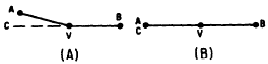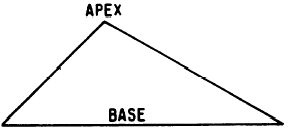Geometric RelationshipsCustom SearchGEOMETRIC RELATIONSHIPS Angles are often classified by their relationship to other angles or to other parts of a geometric figure. For example, angles 1 and 3 inFigure 17-5.-(A) Large obtuse angle; (B) straight angle. figure 17-6 are VERTICAL angles, so called because they share a common vertex. Angles 2 and 4 are opposite each other and are also vertical angles. Lines which cross, as in figure 17-6, always form two pairs of vertical angles, and the vertical angles thus formed are equal in pairs; that is, angle 1 equals angle 3, and angle 2 equals angle 4.Figure 17-6.-Vertical angles. Angles 1 and 2 in figure 17-6 are ADJACENT angles. Other pairs of adjacent angles in figure 17-6 are 2 and 3, 3 and 4, and 1 and 4. In the sense used here, adjacent means side by side, not merely close together or touching. For example, angles 1 and 3 are not adjacent angles even though they touch each other. COMPLEMENTS AND SUPPLEMENTS Two angles whose sum is 90� are complementary. For example, a 60� angle is the complement of a 30� angle, and conversely. "Conversely" is a mathematical word meaning "vice versa." Theo angles whose sum is 180� are supplementary. For example, a 100� angle is the supplement of an 80� angle, and conversely. Practice problems. 1. Describe the angle which is the complement of an acute angle. 2. Describe the angle which is the supplement of a right angle. 3. If two equal angles are complementary, each contains how many degrees? 4. Find the size of an angle which is twice as large as its own complement. (Hint: If x is the angle, then 90� - x is its complement.) Answers: 1. Acute 2. GEOMETRIC FIGURES The discussion of geometric figures in this chapter is limited to polygons and circles. A POLYGON is a plane closed figure, the sides of which are all straight lines. Among the polygons discussed are triangles, parallelograms, and trapezoids. TRIANGLES A triangle is a polygon which has three sides and three angles. In general, any polygon has as many angles as it has sides, and conversely. Parts of a Triangle Each of the three angles of a triangle is a VERTEX; therefore, every triangle has three vertices. The three straight lines joining the vertices are the SIDES (sometimes called legs), and the side upon which the triangle rests is its BASE, often designated by the letter b. This definition assumes that the standard position of a triangle drawn for general discussion is as shown in figure 17-7, in which the triangle is lying on one of its sides. The vertex opposite the base is the highest point of a triangle in standard position, and is thus called the APEX.Figure 17-7.-Triangle in standard position. A straight line perpendicular to the base of a triangle, joining the base to the apex, is the ALTITUDE, often designated by the letter a. The altitude is sometimes referred to as the height, and is then designated by the letter h. Figure 17-8 (B) shows that the apex may not be situated directly above the base. In this case, the base must be extended, as shown by the dashed line, in order to drop a perpendicular from the apex to the base. Mathematicians often use the term "drop a perpendicular." The meaning is the same as "draw a straight, perpendicular line." In general, the geometrical term "distance from a point to a line" means the length of a perpendicular dropped from the point to the line. Many straight lines could be drawn from a line to a point not on the line, but the shortest of these is the one we use in measuring the distance from the point to the line. The shortest one is perpendicular to the line.Figure 17-8.-(A) Interior altitude line; (B) exterior altitude line.Integrated Publishing, Inc. - A (SDVOSB) Service Disabled Veteran Owned Small Business